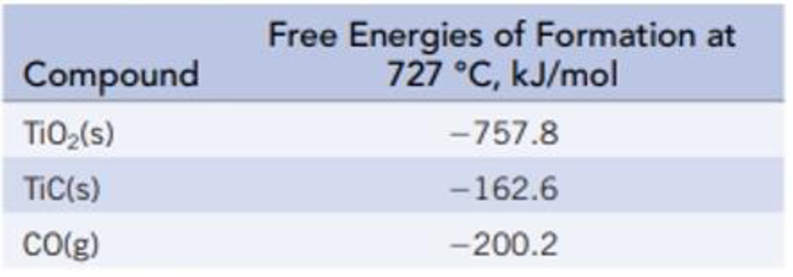Chapter 18, Problem 83IL

Chapter
Section
Textbook Problem

Titanium(IV) oxide is converted to titanium carbide with carbon at a high temperature.TiO2(s) + 3 C(s) → 2 CO(g) + TiC(s)(a) Calculate ΔrG° and K at 727 °C. (b) Is the reaction product-favored at equilibrium at this temperature? (c) How can the reactant or product concentrations be adjusted for the reaction to proceed at 727 °C?

(a)

Interpretation Introduction

Interpretation:

The ΔrGo value and equilibrium constant for the formation of titanium carbide should be calculated.

Concept introduction:

The Gibbs free energy or the free energy change is a thermodynamic quantity represented by ΔrGo. It can be calculated in a similar manner as entropy and enthalpy.  The expression for the free energy change is:

ΔrG°fG°(products)fG°(reactants)

ΔrGo is related to the equilibrium constant Kp by the equation,

ΔrGo=-RTlnKp

The rearranged expression is,

Kp= e-ΔrGoRT

Explanation

The ΔrGo value and the equilibrium constant for the formation of titanium carbide are calculated below.

Given:

The Appendix L referred for the values of standard free energy values.

The given reaction is,

TiO2(s) + 3C(s)2CO(g)+TiC(s)

The ΔrGo for TiO2(s) is 757.8 kJ/mol.

The ΔrGo for C(s) is 0 kJ/mol.

The ΔrGo for CO(g) is 200.2 kJ/mol.

The ΔrGo for TiC(s) is 162.6 kJ/mol.

ΔrG°fG°(products)fG°(reactants)=[[(1 mol TiC(s)/mol-rxn)ΔfG°[TiC(s)]+(2 mol CO(g)/mol-rxn)ΔfG°[CO(g)]]-[(1 mol TiO2(s)/mol-rxn)ΔfG°[TiO2(s)]+(3 mol C(s)/mol-rxn

(b)

Interpretation Introduction

Interpretation:

It should be identified that the reaction will be product favoured at equilibrium at 727oC.

Concept introduction:

The Gibbs free energy or the free energy change is a thermodynamic quantity represented by ΔrGo. It can be calculated in a similar manner as entropy and enthalpy.  The expression for the free energy change is:

ΔrG°fG°(products)fG°(reactants)

ΔrGo is related to the equilibrium constant Kp by the equation,

ΔrGo=-RTlnKp

The rearranged expression is,

Kp= e-ΔrGoRT

(c)

Interpretation Introduction

Interpretation:

The way how the products and reactants for given reaction adjusted in order to proceed at given temperature.

Concept introduction:

The Gibbs free energy or the free energy change is a thermodynamic quantity represented by ΔrGo. It can be calculated in a similar manner as entropy and enthalpy.  The expression for the free energy change is:

ΔrG°fG°(products)fG°(reactants)

ΔrGo is related to the equilibrium constant Kp by the equation,

ΔrGo=-RTlnKp

The rearranged expression is,

Kp= e-ΔrGoRT

Still sussing out bartleby?

Check out a sample textbook solution.

See a sample solution

The Solution to Your Study Problems

Bartleby provides explanations to thousands of textbook problems written by our experts, many with advanced degrees!

Get Started

Find more solutions based on key concepts